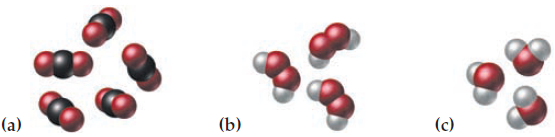×
Get Full Access to Introductory Chemistry - 5 Edition - Chapter 5 - Problem 92p
Get Full Access to Introductory Chemistry - 5 Edition - Chapter 5 - Problem 92p

×

# Specify the number of oxygen atoms (red) represented in each set of molecular models:(a)ISBN: 9780321910295 34

## Solution for problem 92P Chapter 5

Introductory Chemistry | 5th Edition

• Textbook Solutions
• 2901 Step-by-step solutions solved by professors and subject experts
• Get 24/7 help from StudySoup virtual teaching assistantsIntroductory Chemistry | 5th Edition

4 5 1 291 Reviews
18
4
Problem 92P

Specify the number of oxygen atoms (red) represented in each set of molecular models:Step-by-Step Solution:
Step 1 of 3

Solution 92P

Here, we are going to calculate the number of oxygen  atoms present in each  set of molecular models.

Atom is the smallest tiny representation of an atom.

Chemical formula is the representation of the number of atoms present in the molecule or molecular compound .It is model of Carbon dioxide (CO2).

Total number of oxygen atoms in (a) = 2 x 5  =10

(b)It is model of Hydrogen peroxide (H2O2).

Total number of oxygen atoms in (b) = 2 x 3  =6

(c)It is a model of Water molecule (H2O)

Total number of oxygen atoms in (C) = 1 x 3 =3

Step 2 of 3

Step 3 of 3

##### ISBN: 9780321910295

Unlock Textbook Solution